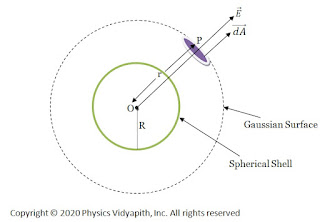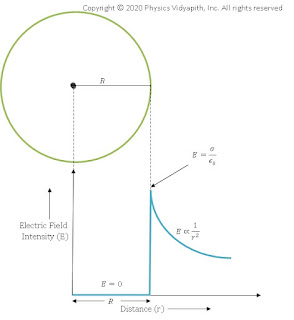### Electric field Intensity due a uniformly charged Spherical Shell

Electric field intensity at a different point in the field due to the uniformly charged spherical shell:

Let us consider, a spherical shell of radius $R$ in which $+q$ charge is distributed uniformly on the surface of the sphere. Now find the electric field intensity at different points due to the spherical shell. These different points are:

1. Electric field intensity at an external point of the spherical shell

2. Electric field intensity on the surface of the spherical shell

3. Electric field intensity at an internal point of the spherical shell

1. Electric field intensity at an external point of the spherical shell:

If point $O$ is the center of the spherical shell, The electric field at the outside of the spherical shell can be determined by the following steps →

1. First, take the point $P$ outside the sphere

2. Draw a spherical surface of radius r which passes through point $P$. This hypothetical surface is known as the Gaussian surface.

3. Now take a small area $\overrightarrow {dA}$ around point $P$ on the Gaussian surface to find the electric flux passing through it.

4. Now find the direction between the electric field vector and small area vector.

Due to uniform charge distribution, the electric field intensity will be the same at every point on the Gaussian surface. So from the figure,Electric field intensity due to the uniformly charged
The direction of electric field intensity on the Gaussian surface is radially outward which is in the direction of the area vector of the Gaussian surface. i.e. ($\theta=0^{\circ}$).Here $\overrightarrow {dA}$ is small area around point $P$ so the small electric flux $d\phi_{E}$ will pass through this small area $\overrightarrow {dA}$. so this flux can be found by applying the Gauss's law in question given below:

$d\phi_{E}= \overrightarrow {E}\cdot \overrightarrow{dA}$

$d\phi_{E}= E\:dA\: cos 0^{\circ} \qquad \left \{\because \theta=0^{\circ} \right \}$

$d\phi_{E}= E\:dA \qquad (1) \quad \left\{\because cos0=1 \right \}$

The electric flux passing through the entire Gaussian surface, So integrate the equation $(1)$ →

$\phi_{E}=\oint E\:dA\qquad(2)$

According to Gauss's law:

$\phi_{E}=\frac{q}{\epsilon_{0}}\qquad (3)$

From equation (1) and equation (2), we can write as

$\frac{q}{\epsilon_{0}}=\oint E\:dA$

$\frac{q}{\epsilon_{0}}= E\oint dA$

The area of entire Gaussian spherical is $\oint {dA}=4\pi r^{2}$. Now substitute this value in above equation. So above equation can be written as:

$\frac{q}{\epsilon_{0}}= E(4\pi r^{2})$

 $E=\frac{1}{4\pi \epsilon_{0}}\frac{q}{r^{2}}$

From the above equation, we can conclude that the behavior of the electric field at the external point due to the uniformly charged spherical shell is the same as, like the entire charge is placed at the center, point charge

If the surface charge density is $\sigma$, Then total charge $q$ on the surface of a spherical shell is →

$q=4\pi R^{2}\: \sigma$

Substitute this value of charge $q$ in above equation, so we can write the equation as:

$E=\frac{1}{4\pi \epsilon_{0}}\frac{4\pi R^{2}\: \sigma}{r^{2}}$

 $E=\frac{\sigma}{\epsilon_{0}}\frac{R^{2}}{r^{2}}$

This equation describes the electric field intensity at the external point of the spherical shell.

2. Electric field intensity on the surface of the spherical shell:

If point $P$ is placed on the surface of the spherical shell i.e ($r=R$). so electric field intensity on the surface of the spherical shell can be found by putting $r=R$ in the formula of the electric field intensity at the external point of the spherical shell:

 $E=\frac{1}{4\pi \epsilon_{0}}\frac{q}{R^{2}}$

 $E=\frac{\sigma}{\epsilon_{0}}$

3. Electric field intensity at an internal point of the spherical shell:

If point $P$ is placed inside the spherical shell then the electric field intensity will be zero at that point because the charge is distributed uniformly on the surface of the solid sphere so there will not be any charge on the Gaussian surface and electric flux will be zero inside the solid sphere. i.e.

$\phi_{E}=\oint E\:dA$

$0=E\oint dA \qquad \left \{ \because \phi_{E}=0 \right \}$

 $E=0$

Electric field intensity distribution with distance for Spherical Shell:

Electric field intensity distribution with distance shows that the electric field is maximum on the surface of the sphere and zero inside the sphere. Electric field intensity distribution outside the sphere reduces with the distance according to $E=\frac{1}{r^{2}}$.Electric field intensity distribution with distance